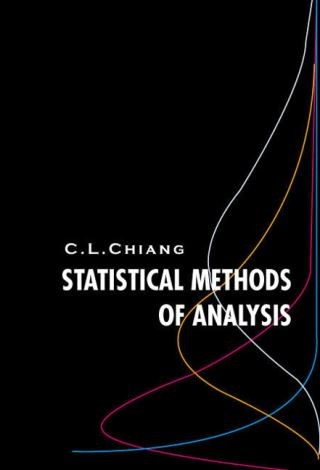## Statistical Methods of Analysis• Description
• Author
• Info
• Reviews

### Description

This textbook systematically presents fundamental methods of statistical analysis: from probability and statistical distributions, through basic concepts of statistical inference, to a collection of methods of analysis useful for scientific research. It is rich in tables, diagrams, and examples, in addition to theoretical justification of the methods of analysis introduced. Each chapter has a section entitled “Exercises and Problems” to accompany the text. There are altogether about 300 exercises and problems, answers to the selected problems are given. A section entitled “Proof of the Results in This Chapter” in each chapter provides interested readers with material for further study.

Contents:
• Descriptive Statistics
• Probability
• Random Variables
• Probability Distributions
• Statistical Inference — Interval Estimation
• Hypothesis Testing — Fundamental Concepts
• Testing Hypotheses Concerning Population Means and Population Proportions
• The Chi-Square Test
• Linear Regression
• Correlation
• Multiple Regression and Correlation
• One-Way Analysis of Variance
• Two-Way Analysis of Variance — Fixed Effects Model
• Two-Way Analysis of Variance Random-Effects Model and Mixed Model
• Design of Experiment
• Analysis of Covariance
• Non-Parametric Statistics

Readership: Instructors and students in applied statistics.